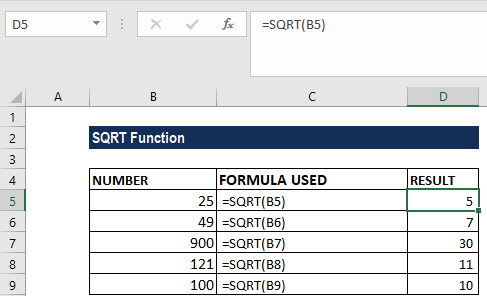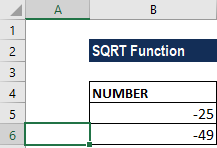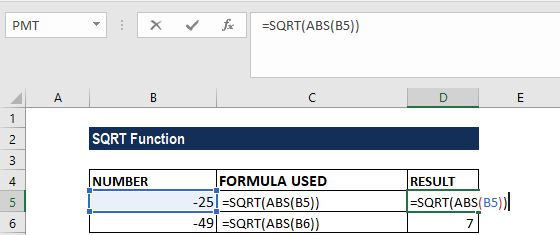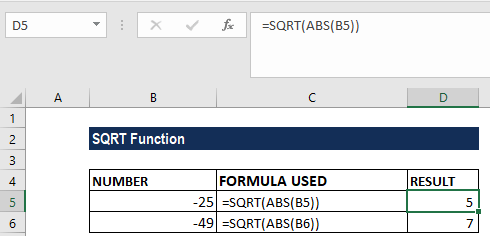# SQRT Function

Returns the square root of a positive number

## What is the SQRT Function?

The SQRT Function is an Excel Math and Trigonometry function. It will provide the square root of a positive number. The function was introduced in MS Excel 2010.

### Formula

=SQRT(number)

The SQRT function uses the following argument:

1. Number (required argument) – This is the number for which we wish to find out the square root. It must be a positive number, an Excel formula, or a function that results in a positive number.

### How to use the SQRT Function in Excel?

To understand the uses of the SQRT function, let’s consider a few examples:

#### Example 1

Suppose we wish to find the square root of the following numbers:

• 25
• 49
• 900
• 121
• 100

The formula used would be =SQRT(reference). We will get the following results:#### Example 2

If a number provided by a user is negative, the SQRT function will return the #NUM! error. The square root of a negative number does not exist among the set of real numbers. It is because there is no way to square a number and get a negative result.

However, if we wish to take the square root of a negative number as if it were a positive number, we need to use the ABS function. We will wrap the source number in the ABS function, which will return the absolute value of a number and ignore the positive or negative sign of the given number:

Suppose we are given the data below:To find the SQRT, we will use the formula =SQRT(ABS(ref)), as shown below:We get the result below:### Things to remember about the SQRT Function

1. #NUM! error – Occurs when the supplied number argument is negative.
2. The SQRT function, depending on the user’s requirement, can be used along with the ABS, ROUND, ROUNDUP, and ROUNDDOWN functions.
3. SQRT is similar to the POWER function. However, the POWER function works like an exponent in a standard math equation. For example, for the number 25, we will provide the formula =SQRT(25) and =POWER( 25, 1/2).

Thanks for reading CFI’s guide to the Excel SQRT function. By taking the time to learn and master these Excel functions, you’ll significantly speed up your financial analysis. To learn more, check out these additional CFI resources:

• Excel Functions for Finance